﻿ 基于倒摆模型的行人行走的稳定性分析

# 基于倒摆模型的行人行走的稳定性分析Stability Analysis of a Pedestrian’s Walking Motion Based on the Inverted Pendulum Model

Abstract: It is essential to understand dynamics of a pedestrian’s walking motion in the study of pedestri-an-structure interaction. In this paper we investigate the stability of a pedestrian’s walking motion by using the inverted pendulum model with harmonic vibrations of the pivot point. Our theoretical research shows that not only the ratio between the lateral and vertical amplitudes, but also the ratio between the lateral and vertical frequencies of the pivot point has significant effects on the balance of the inverted pendulum. Numerical simulations are carried out to verify the correctness of theoretical analysis. Furthermore, according to comparison of stability conditions for different ratios between lateral and vertical frequencies, the reason why the ratio between the lateral and vertical frequencies is closed to 1/2 for a normal pedestrian is exposed. In many pe-destrian-structure problems it is difficult to directly determine the forces induced by pedestrians. Our research indicates that it may be a novel idea by applying the inverted pendulum model to analyze dynamic interaction between pedestrians and a structure, which avoids the difficulty of determination of forces exerted by pedestrians.

1. 引言

2. 倒摆模型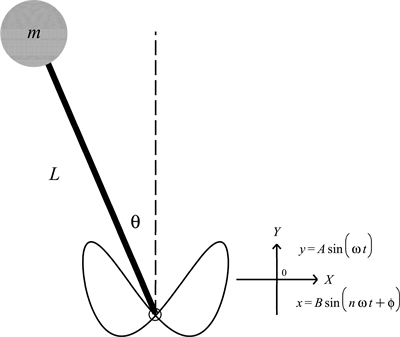Figure 1. The diagram of the inverted pendulum model, in which the pivot point periodically moves up, down, left and right

$\upsilon =\sqrt{{\stackrel{˙}{x}}^{2}-2L\mathrm{cos}\theta \stackrel{˙}{x}\stackrel{˙}{\theta }+{y}^{2}-2L\stackrel{˙}{y}\stackrel{˙}{\theta }\mathrm{sin}\theta +{L}^{2}{\stackrel{˙}{\theta }}^{2}}$(1)

$M=T-V=\frac{1}{2}m{\upsilon }^{2}-mg\left(y+L\mathrm{cos}\theta \right)$(2)

$\frac{\text{d}}{\text{d}t}\frac{\partial M}{\partial \stackrel{˙}{\theta }}-\frac{\partial M}{\partial \theta }=0$(3)

$L\stackrel{¨}{\theta }+\left(-g+A{\omega }^{2}\mathrm{sin}\left(\omega t\right)\right)\mathrm{sin}\theta +B{n}^{2}{\omega }^{2}\mathrm{cos}\left(n\omega t+\varphi \right)\mathrm{cos}\theta =0$(4)

$\stackrel{¨}{\theta }+\left(\delta +\epsilon \mathrm{sin}t\right)\mathrm{sin}\theta +\nu {\epsilon }^{2}\mathrm{sin}\left(nt+\varphi \right)\mathrm{cos}\theta =0$(5)

$\delta =-\frac{g}{L{\omega }^{2}}$$\epsilon =\frac{A}{L}$$\nu =\frac{{n}^{2}BL}{{A}^{2}}$

$\stackrel{¨}{\theta }+\left(\delta +\epsilon \mathrm{sin}t\right)\left(\theta -\frac{1}{6}{\theta }^{3}\right)+\nu {\epsilon }^{2}\mathrm{sin}\left(nt+\varphi \right)\left(1-\frac{1}{2}{\theta }^{2}\right)=0$(6)

3. 倒摆控制方程的通解

$\stackrel{¨}{\theta }+\delta \left(\theta -\frac{{\theta }^{3}}{6}\right)=0$(7)

$\theta \left(t\right)=\epsilon {\theta }_{1}\left(t\right)+{\epsilon }^{2}{\theta }_{2}\left(t\right)+\cdots$(8)

$\begin{array}{l}{\stackrel{¨}{\theta }}_{1}+\delta {\theta }_{1}=0,\\ {\stackrel{¨}{\theta }}_{2}+\delta {\theta }_{2}=-\mathrm{sin}t{\theta }_{1}-\nu \mathrm{sin}\left(nt+\varphi \right).\end{array}$ (9)

(9)中第一式的通解可以写为

${\theta }_{1}=a\mathrm{cos}\left(\omega t\right)+b\mathrm{sin}\left(\omega t\right)$(10)

$\begin{array}{c}{\theta }_{2}=-\frac{1}{2\left(2\omega -1\right)}\left[b\mathrm{cos}\left(\left(\omega -1\right)t\right)-a\mathrm{sin}\left(\left(\omega -1\right)t\right)\right]-\frac{\nu \mathrm{sin}\left(nt+\varphi \right)}{{\omega }^{2}-{n}^{2}}\\ -\frac{1}{2\left(2\omega +1\right)}\left[b\mathrm{cos}\left(\left(\omega +1\right)t\right)-a\mathrm{sin}\left(\left(\omega +1\right)t\right)\right].\end{array}$ (11)

$\delta >0$ 时(6)的二阶近似解可以写为

$\begin{array}{c}\theta =\epsilon \left(a\mathrm{cos}t+b\mathrm{sin}t\right)+{\epsilon }^{2}\left\{-\frac{1}{2\left(2\omega -1\right)}\left[b\mathrm{cos}\left(\left(\omega -1\right)t\right)-a\mathrm{sin}\left(\left(\omega -1\right)t\right)\right]\\ -\frac{1}{22\left(2\omega +1\right)}\left[b\mathrm{cos}\left(\left(\omega +1\right)t\right)-a\mathrm{sin}\left(\left(\omega +1\right)t\right)\right]-\frac{\nu \mathrm{sin}\left(nt+\varphi \right)}{{\omega }^{2}-{n}^{2}}\right\}\end{array}$ (12)(a) $\delta =0.02$ , $\epsilon =0.01$ , $\nu =0.1$ , $n=0.1$ , $\varphi =0.1$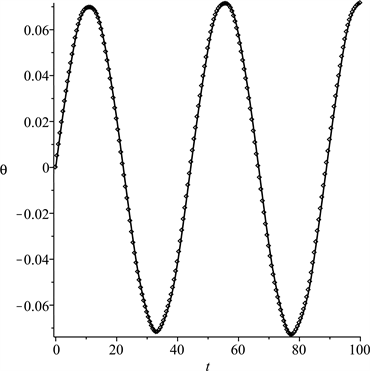(b) $\delta =0.05$ , $\epsilon =0.03$ , $\nu =0.2$ , $n=0.2$ , $\varphi =0.2$(c) $\delta =0.08$ , $\epsilon =0.05$ , $\nu =0.3$ , $n=0.7$ , $\varphi =0.3$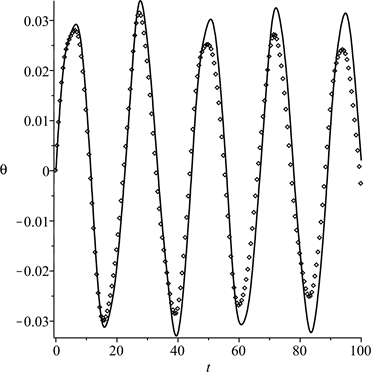(d) $\delta =0.1$ , $\epsilon =0.07$ , $\nu =0.4$ , $n=0.8$ , $\varphi =0.4$

Figure 2. Comparison between analytical solution (···dot line) and the numerical solution (— solid line)

4. 分界线和周期解

$\delta ={\delta }_{0}+{\delta }_{1}\epsilon +{\delta }_{2}{\epsilon }^{2}+\cdots$(13)

${\stackrel{¨}{\theta }}_{1}+{\delta }_{0}{\theta }_{1}=0$(14)

${\stackrel{¨}{\theta }}_{2}+{\delta }_{0}{\theta }_{2}=-\left({\delta }_{1}+\mathrm{sin}t\right){\theta }_{1}-\nu \mathrm{sin}\left(nt+\varphi \right)$(15)

${\stackrel{¨}{\theta }}_{3}+{\delta }_{0}{\theta }_{3}=-\left({\delta }_{1}+\mathrm{sin}t\right){\theta }_{2}-\left({\delta }_{2}{\theta }_{1}-\frac{1}{6}{\delta }_{0}{\theta }_{1}^{3}\right)$(16)

4.1. δ0 = 0

${\delta }_{0}=0$ 带入(14)，有 ${\theta }_{1}=a$ ，其中 $a$ 为常数依赖初始条件。此时(15)变为

${\stackrel{¨}{\theta }}_{2}+a\left({\delta }_{1}+\mathrm{sin}t\right)+\nu \mathrm{sin}\left(nt+\varphi \right)=0$(17)

${\theta }_{2}=a\mathrm{sin}t+\frac{\nu }{{n}^{2}}\mathrm{sin}\left(nt+\varphi \right)$(18)

${\theta }_{3}=-\frac{a}{8}\mathrm{cos}\left(2t\right)+\frac{\nu \mathrm{cos}\left[\left(n-1\right)t+\varphi \right]}{2{n}^{2}{\left(n-1\right)}^{2}}-\frac{\nu \mathrm{cos}\left[\left(n+1\right)t+\varphi \right]}{2{n}^{2}{\left(n+1\right)}^{2}}.$ (19)

$\delta =-\frac{1}{2}{\epsilon }^{2}$(20)

$\theta =a\epsilon +\left[a\mathrm{sin}t+\frac{\nu }{{n}^{2}}\mathrm{sin}\left(nt+\varphi \right)\right]{\epsilon }^{2}+\left[-\frac{1}{8}a\mathrm{cos}\left(2t\right)+\frac{\nu \mathrm{cos}\left(\left(n-1\right)t+\varphi \right)}{2{n}^{2}{\left(n-1\right)}^{2}}-\frac{\nu \mathrm{cos}\left(\left(n+1\right)t+\varphi \right)}{2{n}^{2}{\left(n+1\right)}^{2}}\right]{\epsilon }^{3}.$ (21)

4.2. δ0 = n2 (n ≠ 1/2)

${\delta }_{0}={n}^{2}$ 带入(14)，得到(14)的通解

${\theta }_{1}=a\mathrm{cos}\left(nt\right)+b\mathrm{sin}\left(nt\right)$(22)

${\stackrel{¨}{\theta }}_{2}+{n}^{2}{\theta }_{2}=-\left({\delta }_{1}+\mathrm{sin}t\right)\left[a\mathrm{cos}\left(nt\right)+b\mathrm{sin}\left(nt\right)\right]-\nu \mathrm{sin}\left(nt+\varphi \right)$(23)

${\theta }_{2}$ 为周期解，必须要消去久期项，因此有

$\begin{array}{l}\nu \mathrm{cos}\left(\varphi \right)+b{\delta }_{1}=0,\\ \nu \mathrm{sin}\left(\varphi \right)+a{\delta }_{1}=0.\end{array}$ (24)

a，b是任意依赖初始条件的常数，(24)的非平凡解可以写为

$a=0,\varphi =0,{\delta }_{1}=-\frac{\nu }{b},$

$b=0,\varphi =\frac{\text{π}}{2},{\delta }_{1}=-\frac{\nu }{a}.$

4.2.1. $a=0,\varphi =0,{\delta }_{1}=-\frac{\nu }{b},$

${\theta }_{2}=-\frac{b\mathrm{cos}\left(\left(n-1\right)t\right)}{2\left(2n-1\right)}-\frac{b\mathrm{cos}\left(\left(n+1\right)t\right)}{2\left(2n+1\right)}.$ (25)

$\begin{array}{l}{\delta }_{2}=\frac{4{b}^{2}{n}^{4}-{b}^{2}{n}^{2}+4}{8\left(4{n}^{2}-1\right)},\\ {\theta }_{3}=-\frac{\nu \mathrm{cos}\left(\left(n-1\right)t\right)}{2{\left(2n-1\right)}^{2}}+\frac{\nu \mathrm{cos}\left(\left(n+1\right)t\right)}{{\left(2n+1\right)}^{2}}+\frac{{b}^{3}}{192}\mathrm{sin}\left(3nt\right)-\frac{b\mathrm{sin}\left(\left(n-2\right)t\right)}{16\left(2n-1\right)\left(n-1\right)}-\frac{b\mathrm{sin}\left(\left(n+2\right)t\right)}{16\left(2n+1\right)\left(n+1\right)}.\end{array}$ (26)

$\delta ={n}^{2}-\frac{\nu }{b}\epsilon +\frac{4{b}^{2}{n}^{4}-{b}^{2}{n}^{2}+4}{8\left(4{n}^{2}-1\right)}{\epsilon }^{2}.$ (27)

4.2.2. $b=0,\varphi =\pi /2,{\delta }_{1}=-\nu /a$

$\delta ={n}^{2}-\frac{\nu }{a}\epsilon +\frac{4{a}^{2}{n}^{4}-{a}^{2}{n}^{2}+4}{8\left(4{n}^{2}-1\right)}{\epsilon }^{2},$ (28)

$\begin{array}{c}\theta =a\mathrm{cos}\left(nt\right)\epsilon +\left[\frac{a\mathrm{sin}\left(\left(n-1\right)t\right)}{2\left(2n-1\right)}+\frac{a\mathrm{sin}\left(\left(n+1\right)t\right)}{2\left(2n+1\right)}\right]{\epsilon }^{2}+\left[\frac{\nu \mathrm{sin}\left(\left(n-1\right)t\right)}{2{\left(2n-1\right)}^{2}}\\ -\frac{\nu \mathrm{sin}\left(\left(n+1\right)t\right)}{2{\left(2n+1\right)}^{2}}-\frac{a\mathrm{cos}\left(\left(n-2\right)t\right)}{\left(2n-1\right)\left(n-1\right)}-\frac{a\mathrm{cos}\left(\left(n+2\right)t\right)}{\left(2n+1\right)\left(n+1\right)}-\frac{{a}^{3}}{192}\mathrm{cos}\left(3nt\right)\right]{\epsilon }^{3}.\end{array}$ (29)

4.3. δ0 = 1/4

4.3.1. a = b

$\delta =\frac{1}{4}-\frac{1}{2}\epsilon +\left(\frac{{b}^{2}}{16}-\frac{1}{8}\right){\epsilon }^{2},$ (30)

$\begin{array}{c}\theta =b\left[\mathrm{cos}\left(\frac{1}{2}t\right)+\mathrm{sin}\left(\frac{1}{2}t\right)\right]\epsilon +\frac{1}{4}\left[-b\mathrm{cos}\left(-\frac{3}{2}t\right)+b\mathrm{sin}\left(-\frac{3}{2}t\right)+\frac{16\nu \mathrm{sin}\left(nt+\varphi \right)}{4{n}^{2}-1}\right]{\epsilon }^{2}\\ +\left[\frac{b\left({b}^{2}+6\right)}{96}\left(\mathrm{cos}\left(-\frac{3}{2}t\right)+\mathrm{sin}\left(-\frac{3}{2}t\right)\right)-\frac{b}{48}\left(\mathrm{cos}\left(\frac{5}{2}t\right)+\mathrm{sin}\left(\frac{5}{2}t\right)\right)\\ +\frac{8\nu \mathrm{cos}\left(\left(n-1\right)t+\varphi \right)}{\left(2n+1\right)\left(2n-3\right){\left(2n-1\right)}^{2}}-\frac{8\nu \mathrm{cos}\left(\left(n+1\right)t+\varphi \right)}{\left(2n-1\right)\left(2n+3\right){\left(2n+1\right)}^{2}}-\frac{8\nu \mathrm{sin}\left(nt+\varphi \right)}{{\left(4{n}^{2}-1\right)}^{2}}\right]{\epsilon }^{3}.\end{array}$ (31)

4.3.2. a = −b

$\delta =\frac{1}{4}\text{+}\frac{1}{2}\epsilon +\left(\frac{{b}^{2}}{16}-\frac{1}{8}\right){\epsilon }^{2},$ (32)

$\begin{array}{c}\theta =b\left[-\mathrm{cos}\left(\frac{1}{2}t\right)+\mathrm{sin}\left(\frac{1}{2}t\right)\right]\epsilon -\frac{1}{4}\left[b\mathrm{cos}\left(\frac{3}{2}t\right)+b\mathrm{sin}\left(\frac{3}{2}t\right)-\frac{16\nu \mathrm{sin}\left(nt+\varphi \right)}{4{n}^{2}-1}\right]{\epsilon }^{2}\\ -\left[\frac{b\left({b}^{2}+6\right)}{96}\left(\mathrm{cos}\left(\frac{3}{2}t\right)+\mathrm{sin}\left(\frac{3}{2}t\right)\right)+\frac{b}{48}\left(\mathrm{cos}\left(\frac{5}{2}t\right)+\mathrm{sin}\left(\frac{5}{2}t\right)\right)\\ +\frac{8\nu \mathrm{cos}\left(\left(n-1\right)t+\varphi \right)}{\left(2n+1\right)\left(2n-3\right){\left(2n-1\right)}^{2}}-\frac{8\nu \mathrm{cos}\left(\left(n+1\right)t+\varphi \right)}{\left(2n-1\right)\left(2n+3\right){\left(2n+1\right)}^{2}}+\frac{8\nu \mathrm{sin}\left(nt+\varphi \right)}{{\left(4{n}^{2}-1\right)}^{2}}\right]{\epsilon }^{3}.\end{array}$ (33)

5. 稳定性区域分析和讨论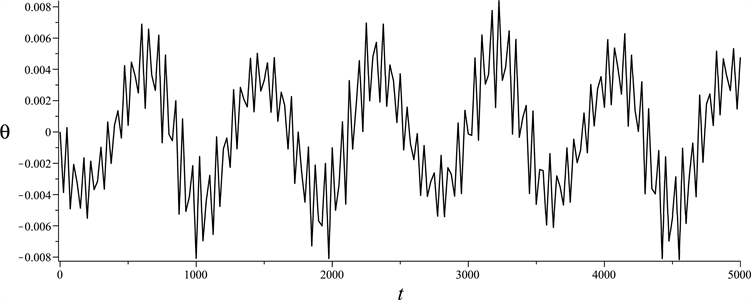(a) The history time curves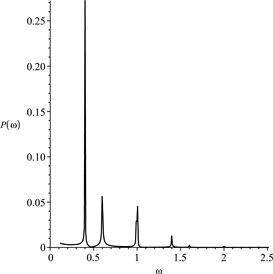(b) The phase-plane trajectory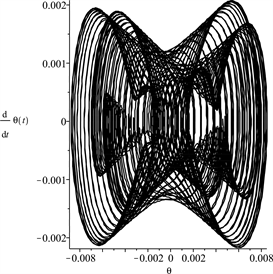(c) The Fourier power spectrum

Figure 3. The direct numerical results for Equation (6) with δ = −0.02, $\epsilon =0.2$ , $\nu =0.01$ , n = 0.4 and $\varphi =0.15$ , the initial conditions are taken as $\theta \left(0\right)=0$ and $\stackrel{˙}{\theta }\left(0\right)=0.001$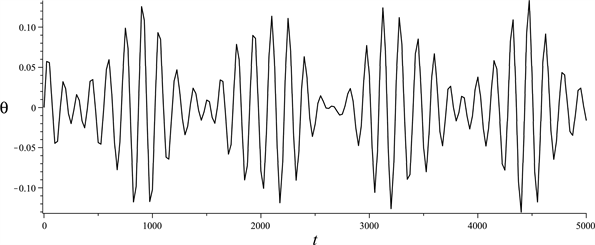(a) The history time curves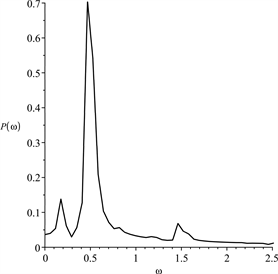(b) The phase-plane trajectory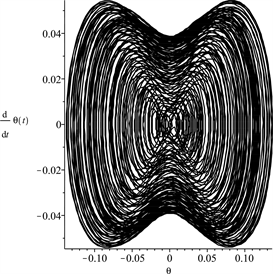(c) The Fourier power spectrum

Figure 4. The direct numerical results for Equation (6) with δ = −0.02, $\epsilon =0.482$ , $\nu =0.01$ , n = 0.8 and $\varphi =0.15$ , the initial conditions are taken as $\theta \left(0\right)=0$ and $\stackrel{˙}{\theta }\left(0\right)=0.001$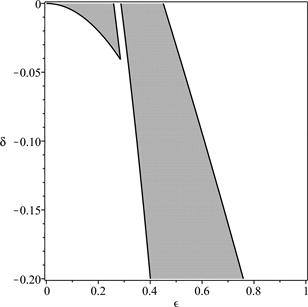(a) n = 0.25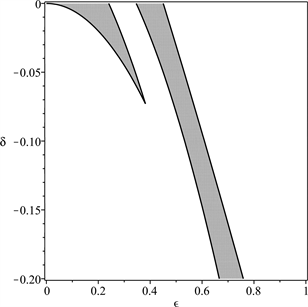(b) n = 0.45(c) n = 0.55(d) n = 0.75

Figure 5. The stable regions (marked with grey shades) of solutions of Equation (6) under the conditions $\epsilon >0$ and δ < 0, in which $\nu =0.01$ , $\varphi =0.15$ , a = 0.1, b = −0.2

$\nu$ 是倒摆支点水平和竖向振幅之比。为分析 $\nu$ 对稳定性区域的影响，分别令 $\nu$ 取0.05，0.1，0.15。其它参数和图5中参数保持一致。 $\nu$ 对稳定区域的影响如图7所示。从图5图7可以看出，当 $n<1/2$$\nu$ 对稳定性区域有很大的影响；当 $n>1/2$$\nu$ 的变化几乎没有影响。当 $\nu$ 很小并接近0时， $n<1/2$ 时的稳定性区域的面积可能超过 $n>1/2$ 时，但 $n<1/2$ 时稳定性区域是不连续的(图5(b))。当 $n<1/2$ 时，随着 $\nu$ 的增加，稳定性区域的不连续性开始消失，稳定性区域的面积显著下降。对比图5图7$\nu$ 的增加总是使得不稳定性增加，此时不管n值的大小，通过减小 $\epsilon$ 和δ来增加稳定性总是有效的。根据图5~7的分析对倒摆系统如果要获得好的稳定性需要 $n>1/2$(a) n = 0.25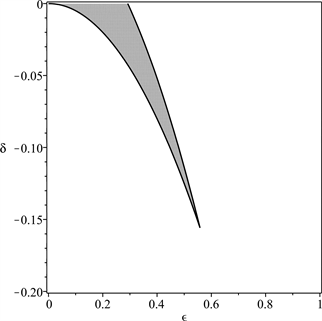(b) n = 0.45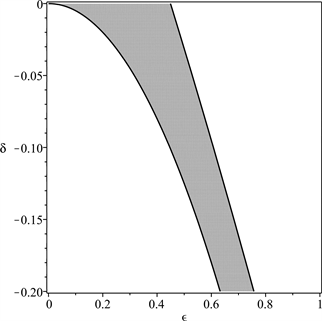(c) n = 0.55(d) n = 0.75

Figure 6. The stable regions (marked with grey shades) of solutions of Equation (6) under the conditions $\epsilon >0$ and δ < 0, in which $\nu =0.01$ , $\varphi =0.15$ , a = 0.5, b = −0.01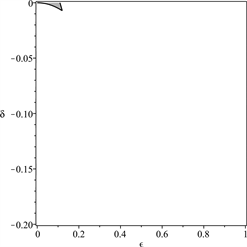(a) $\nu =0.05$ , n=0.25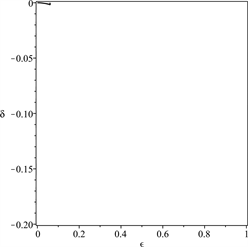(b) $\nu =0.1$ , n=0.25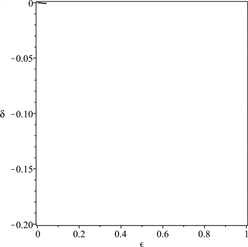(c) $\nu =0.15$ , n=0.25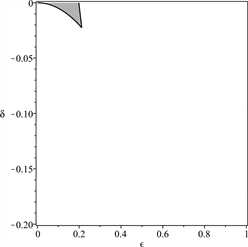(d) $\nu =0.05$ , n=0.45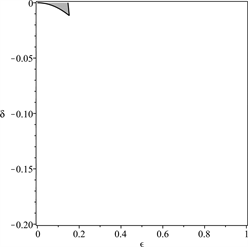(e) $\nu =0.1$ , n=0.45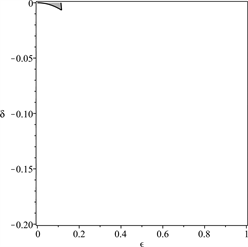(f) $\nu =0.15$ , n=0.45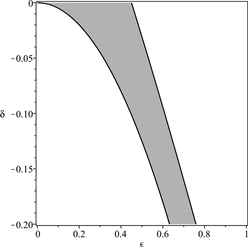(g) $\nu =0.05$ , n=0.55(h) $\nu =0.1$ , n=0.55(i) $\nu =0.15$ , n=0.55(j) $\nu =0.05$ , n=0.75(k) $\nu =0.1$ , n=0.75(l) $\nu =0.15$ , n=0.75

Figure 7. The stable regions (marked with grey shades) of solutions of Equation (6) under the conditions $\epsilon >0$ and δ < 0, in which $\varphi =0.15$ , a = 0.1 and b = −0.2

6. 结论

 Ehsan, A., Colin, C., Stana, Z., Neil, E. and Amin, H. (2018) A Framework for Quantification of Human-Structure In-teraction in Vertical Direction. Journal of Sound and Vibration, 432, 351-372.

 Van Nimmen, K., Lombaert, G., De Roeck, G. and Van den Broeck, P. (2017) The Impact of Vertical Human-Structure Interaction on the Response of Footbridges to Pedestrian Excitation. Journal of Sound and Vibration, 402, 104-121.
https://doi.org/10.1016/j.jsv.2017.05.017

 Maria, G.M., Eleonora, L. and Giulia, L. (2018) Coupled Analysis of Footbridge-Pedestrian Dynamic Interaction. Engineering Structures, 176, 127-142.

 Dallard, P., Fitzpatrick, A.J., Flint, A., Le Bourva, S., Low, A., Ridsdill Smith, R.M. and Willford, M. (2001) The London Millennium Footbridge. Structure Engineering, 79, 17-33.

 Nakamura, S. and Kawasaki, T. (2009) A Method for Predicting the Lateral Girder Response of Footbridges Induced by Pedestrian. Journal of Constructional Steel Research, 65, 1705-1711.
https://doi.org/10.1016/j.jcsr.2009.03.003

 Roberts, T.M. (2005) Lateral Pedestrian Excitation of Footbridges. Journal of Bridge Engineering, 10, 107-112.
https://doi.org/10.1061/(ASCE)1084-0702(2005)10:1(107)

 Erfan, S., Aleksandar, P. and Vitomir, R. (2017) Structural Vibration Serviceability: New Design Framework Featuring Human-Structure Interaction. Engineering Structures, 136, 295-311.

 Xinxin, W., Van den Broeck, P., Guido, D.R. and Katrien, V.N. (2017) A Simplified Method to Account for the Effect of Human-Human Interaction on the Pedestrian-Induced Vibrations of Footbridges. Procedia Engineering, 199, 2907-2912.
https://doi.org/10.1016/j.proeng.2017.09.331

 Zhou, D., Han, H., Ji, T. and Xu, X. (2016) Comparison of Two Models for Human-Structure Interaction. Applied Mathematical Modelling, 40, 3738-3748.
https://doi.org/10.1016/j.apm.2015.10.049

 Macdonald, J.H.G. (2008) Lateral Excitation of Bridges by Balancing Pedestrians. Proceedings of the Royal Society of London A: Mathematical, Physical and Engi-neering Sciences, 465, 1055-1073.

 De, H.M., Geurts, A.C., Dault, M.C., et al. (2005) Restoration of Weight-Shifting Capacity in Patients with Postacute Stroke: A Rehabilitation Cohort Study. Archives of Physical Med-icine & Rehabilitation, 86, 755-762.
https://doi.org/10.1016/j.apmr.2004.10.010

 Maurer, C. and Peterka, R.J. (2005) A New Interpretation of Spontaneous Sway Measures Based on a Simple Model of Human Postral Control. Journal of Neurophysiology, 93, 189-200.
https://doi.org/10.1152/jn.00221.2004

 Erlicher, S., Trovato, A. and Argoul, P. (2010) Modeling the Lateral Pedestrian Force on a Rigid Floor by a Self-Sustained Oscillator. Mechanical System and Signal Processing, 24, 1579-1604.
https://doi.org/10.1016/j.ymssp.2009.11.006

Top